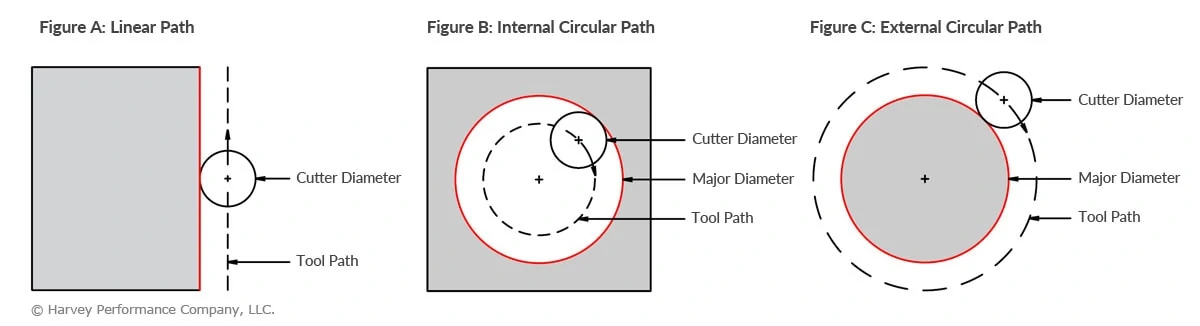## Circular Interpolation: Machining Circular Tool Paths

When machining, proper speeds and feeds are very important to avoid breakage and maximize performance. Traditional end milling formulas use Surface Footage (SFM) and Chip Load (IPT) to calculate Speed (RPM) and Feed (IPM) rates. These formulas dictate the correct machining parameters for use in a linear path in which the end mill’s centerline is travelling in a straight line. Since not all parts are made of flat surfaces, end mills will invariably need to move in a non-linear path. In the case of machining circular tool paths, the path of the end mill’s centerline is circular. Not surprisingly, this is referred to as Circular Interpolation.

## Cutting Circular Tool Paths

All rotating end mills have their own angular velocity at the outside diameter. But when the tool path is circular, there is an additional component that is introduced, resulting in a compound angular velocity. Basically, this means the velocity of the outside diameter is travelling at a substantially different velocity than originally expected. The cause of the compound angular velocity is seen in the disparity between the tool path lengths.

### Internal Circular Tool Paths

Figure A shows the cross section of a cutting tool on a linear path, with the teeth having angular velocity due to tool rotation, and the center of the tool having a linear feed. Note that the tool path length will always be equal to the length of the machined edge. Figure B shows the same cutting tool on an internal circular path, as done when machining a hole. In this case, the angular velocity of the teeth is changed as a result of an additional component from the circular path of the tool’s center. The diameter of the tool path is smaller than that of the major diameter being cut. Or, in other words, the tool path length is shorter than the machined edge length, increasing the angular velocity of the teeth. To prevent overfeeding and the possibility of tool breakage, the increased angular velocity of the teeth must be made the same as in the linear case in Figure A. The formula below can be used to properly lower the feed rate for internal machining:

Internal Adjusted Feed = (Major Diameter-Cutter Diameter) / (Major Diameter) × Linear Feed### External Circular Tool Paths

Figure C shows the same cutting tool on an external circular path, as done when machining a post. In this case, the diameter of the tool path is larger than the major diameter being cut. This means that the tool path length is longer than the machined edge length, resulting in a decreased angular velocity. To prevent premature dulling and poor tool life due to over-speeding, use the formula below to properly raise the feed rate for external machining. In this way, the decreased angular velocity of the teeth is made the same as in the linear case in Figure A.

External Adjusted Feed = (Major Diameter+Cutter Diameter) / (Major Diameter) × Linear Feed